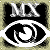About Store Forum Documentation ContactHelp, how to rotate a mesh in a certain way... Author Message
jordicatMember

 Post: #1 Help, how to rotate a mesh in a certain way... Hi comunity, can someone to help me? I am trying to do this mathematical things: 1-I have balls (or spheres). Between each pair of balls I have to put a connection (a long cylinder mesh with a small radius) that connects a pair of balls. Each cylinder has vertical orientation (0,-1,0). 2-I have to create 2 matrices. One matrix position to put the cylinder at the center of one of the pair of balls, and a matrix rotation to rotate this cylinder to connect this pair of balls. The cylinder has distance=Dist(center ball1, center ball2). 3-to do this I have this code: void IniPosRotCylinder(int iball1, int iball2, int icylinder) { float uv, modu, modv, angle, cos; Vec u(0, 1, 0),v,uxv; // u=vector initial cylinder with no rotation //---------- calcule angle vectors u,v ----------------------- v = Gl.c[iball2].pos - Gl.c[iball1].pos; v.normalize(); uv = Dot(u, v); // dotproduct = u.x * v.x + u.y * v.y + u.z * v.z; modu = 1; modv = 1; // modulus of u,v (directly) cos = uv / (modu * modv); angle = Acos(cos); // in radians // get normal to plane u,v uxv = Cross(u, v); uxv.normalize(); //--- calculate matrix position of cylinder Matrix m1(MatrixIdentity); Vec pos = Gl.c[iball1].pos; // ball pos m1.setPos(pos); Matrix m2(MatrixIdentity); m2.setRotate(uxv, angle); //--- Put cylinder to matrix position m1 and rotate by matrix m2 Gl.cnx[icylinder].mt = m2*m1; } this picture try to explain this:In a other 3D engine (TV3D) I could make this with the function:But I want to do this with Esenthel engine. I suppose that I need to keep in mind the center position of cylinder before I put to it exactly at center ball (p1). Note: u=initial cylinder vector rotation, v=vector between p2-p1. I am sure it's not easy to do, but I did this in the other engine. In Esenthel perhaps is possible to do this in a other way, perhaps in a few functions. I hope with the pictures the problem is understandable. Thanks very much for your attention.
12-06-2009 05:46 PM
EsenthelPost: #2 RE: Help, how to rotate a mesh in a certain way... you can use Matrix().setPosDir or setPosUp
12-06-2009 09:23 PM
jordicatMember

 Post: #3 RE: Help, how to rotate a mesh in a certain way... Thanks, I have used instead this code: Vec v2 = (Gl.c[ibola2].pos-Gl.c[ibola1].pos) / Vec(2); Matrix m1(MatrixIdentity); m1.setPos(Gl.c[ibola2].pos-v2); Matrix m2(MatrixIdentity); m2.setRotation(Vec(0,0,0), u, v); Gl.cnx[iconex].mt = m2*m1; and it works perfect.Another thing. I can not type many keys in the message box with my spanish-catalan keyword correctly. for example when I press key shift+8 to type "(" instead appears "¿" character.
12-06-2009 11:38 PM
MasterxiloMemberPost: #4 RE: Help, how to rotate a mesh in a certain way... Looks like the engine doesn't use the WM_CHAR message sent by the os. Because this would take into account different keyboard layouts... System: Windows 7 Ultimate 64 bit, Q6600 2.4 GHZ, 4GB RAM, nVidia GeForce 260 GTX 896 MB DDR3 Visit my site: hurricane-eye.webs.com And read my blog: hurricane-eyeent.blogspot.com
12-07-2009 12:04 AM
Esentheljordicat